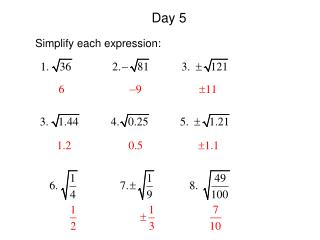DownloadDownload PresentationDay 5

# Day 5

Download Presentation## Day 5

- - - - - - - - - - - - - - - - - - - - - - - - - - - E N D - - - - - - - - - - - - - - - - - - - - - - - - - - -
##### Presentation Transcript

1. Day 5 Simplify each expression:

2. 10.3 Solving Quadratic Equations I can solve quadratic equations by graphing. I can solve quadratic equations by using square roots.

3. 10.3 Solving Quadratic Equations A quadratic equation is an equation that can be written in the form , where a 0. This form is called the standard form of a quadratic equation. A quadratic can have 2, 1, or no real solutions. Solutions of a quadratic equation are called roots of the equation or zeros of the function or x-intercepts.

4. Solving Quadratics by Graphing Graph the following and determine their solutions. Remember: Solutions are where the graph crosses the x-axis. There can be 2, 1, or none. Ex. 1 x y – 2 – 1 0 1 2 0 – 3 – 4 – 3 0

5. Solving Quadratics by Graphing Ex. 2 – 2 – 1 0 1 2 4 1 0 1 4 x y

6. Solving Quadratics by Graphing Ex. 3 – 2 – 1 0 1 2 8 5 4 5 8 x y

7. Solving Quadratics by Using Square Roots Solve the following for x. Ex. 4 Add 98 to both sides. Divide both sides by 2. Square root both sides. Remember: When you square root something, it gives you a plus and a minus answer.

8. Solving Quadratics by Using Square Roots Solve each equation. Ex. 5 Ex. 6 Ex. 7 • Can’t take the square • root of a negative.